# 十一、线性回归

## 添加交互项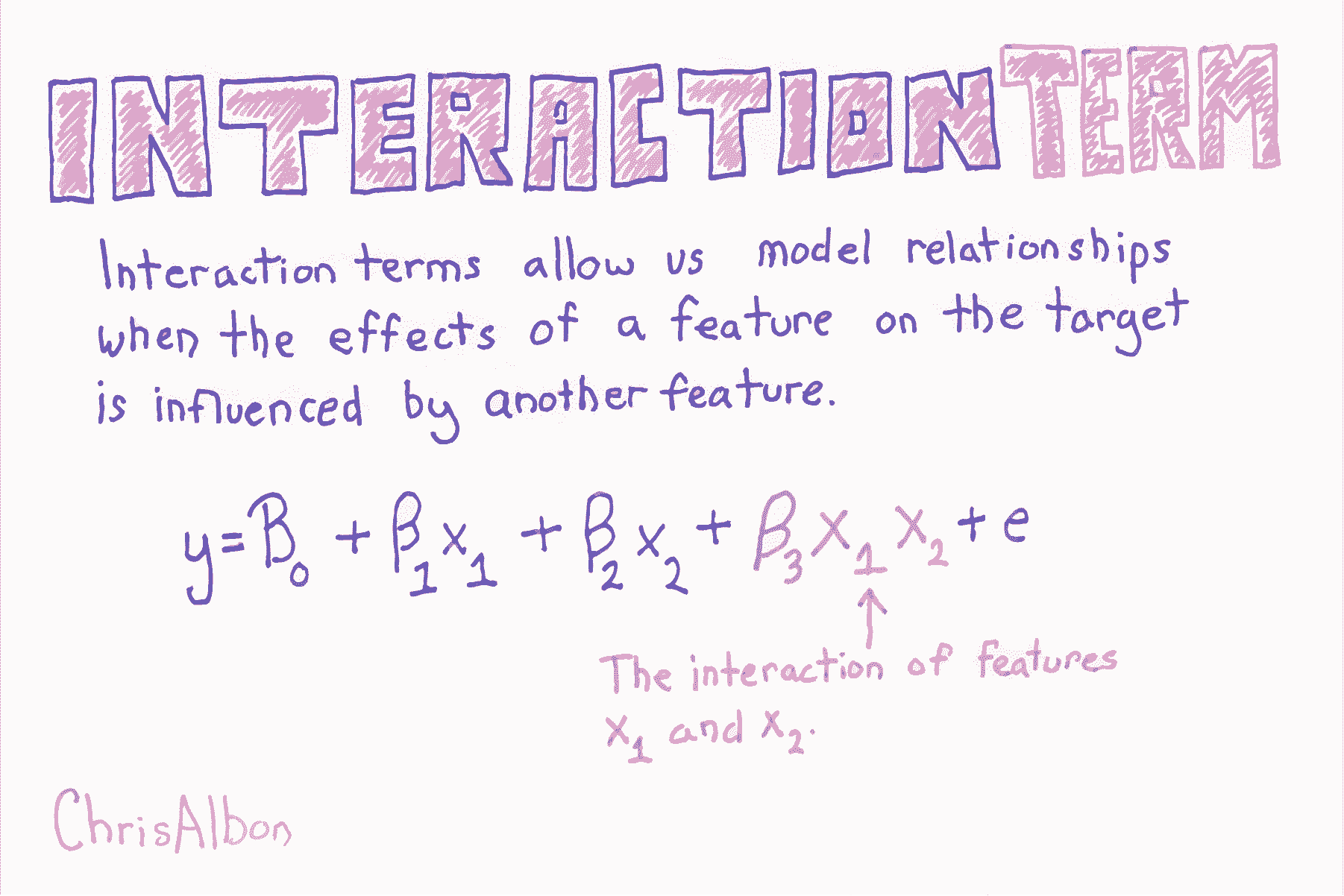``````# 加载库
from sklearn.linear_model import LinearRegression
from sklearn.preprocessing import PolynomialFeatures
import warnings

# 屏蔽警告
warnings.filterwarnings(action="ignore", module="scipy", message="^internal gelsd")

# 加载只有两个特征的数据
X = boston.data[:,0:2]
y = boston.target
````````````# 创建交互项（非多项式特征）
interaction = PolynomialFeatures(degree=3, include_bias=False, interaction_only=True)
X_inter = interaction.fit_transform(X)

# 创建线性回归
regr = LinearRegression()

# 拟合线性回归
model = regr.fit(X_inter, y)
``````

## 创建交互特征

``````# 加载库
from sklearn.preprocessing import PolynomialFeatures
import numpy as np

# 创建特征矩阵
X = np.array([[2, 3],
[2, 3],
[2, 3]])

# 创建 PolynomialFeatures 对象，它的 interaction_only 设为 True
interaction = PolynomialFeatures(degree=2, interaction_only=True, include_bias=False)

# 转换特征矩阵
interaction.fit_transform(X)
'''
array([[ 2.,  3.,  6.],
[ 2.,  3.,  6.],
[ 2.,  3.,  6.]])
'''
``````

## Lasso 回归的 Alpha 的效果

Lasso 回归)是正则化的常用建模技术。 它背后的数学非常有趣，但实际上，你需要知道的是，Lasso 回归带有一个参数`alpha`，而`alpha`越高，大多数特征系数越会为零。

``````from sklearn.linear_model import Lasso
from sklearn.preprocessing import StandardScaler
import pandas as pd

scaler = StandardScaler()
X = scaler.fit_transform(boston["data"])
Y = boston["target"]
names = boston["feature_names"]

# 创建函数 lasso
def lasso(alphas):
'''
接受 alpha 列表。输出数据帧，包含每个 alpha 的 Lasso 回归的系数。
'''
# 创建空数据帧
df = pd.DataFrame()

# 创建特征名称列
df['Feature Name'] = names

# 对于每个列表中的 alpha 值，
for alpha in alphas:
# 创建这个 alpha 值的 laaso 回归，
lasso = Lasso(alpha=alpha)

# 拟合 lasso 回归
lasso.fit(X, Y)

# 为这个 alpha 值创建列名称
column_name = 'Alpha = %f' % alpha

# 创建系数列
df[column_name] = lasso.coef_

# 返回数据帧
return df

# 调用函数 lasso
lasso([.0001, .5, 10])
``````
Feature Name Alpha = 0.000100 Alpha = 0.500000 Alpha = 10.000000
0 CRIM -0.920130 -0.106977 -0.0
1 ZN 1.080498 0.000000 0.0
2 INDUS 0.142027 -0.000000 -0.0
3 CHAS 0.682235 0.397399 0.0
4 NOX -2.059250 -0.000000 -0.0
5 RM 2.670814 2.973323 0.0
6 AGE 0.020680 -0.000000 -0.0
7 DIS -3.104070 -0.169378 0.0
9 TAX -2.074110 -0.000000 -0.0
10 PTRATIO -2.061921 -1.599574 -0.0
11 B 0.856553 0.545715 0.0
12 LSTAT -3.748470 -3.668884 -0.0

# Lasso 回归

``````# 加载库
from sklearn.linear_model import Lasso
from sklearn.preprocessing import StandardScaler

# 加载数据
X = boston.data
y = boston.target

# 标准化特征
scaler = StandardScaler()
X_std = scaler.fit_transform(X)
``````

``````# 创建带有某个 alpha 值的 Lasso
regr = Lasso(alpha=0.5)

# 拟合 Lasso 回归
model = regr.fit(X_std, y)
``````

## 线性回归

``````import pandas as pd
from sklearn import linear_model
import random
import numpy as np
%matplotlib inline
``````

``````# 加载数据

# 打乱数据的行（这是必要的，
# 仅仅由于我使用 DrawMyData 创建数据的方式。真正的分析中通常不需要
df = df.sample(frac=1)
``````

``````# 查看数据的前几行
``````
friendly_battledeaths enemy_battledeaths
7 8.2051 9.6154
286 88.7179 86.1538
164 14.3590 8.8462
180 38.9744 36.5385
89 93.0769 93.0769

``````# 绘制两个变量，彼此对照
df.plot(x='friendly_battledeaths', y='enemy_battledeaths', kind='scatter')

# <matplotlib.axes._subplots.AxesSubplot at 0x1145cdb00>
``````### 步骤

1. 将数据集划分为两个数据集：我们将用于训练模型的“训练”数据集，和我们将用于判断该模型准确率的“测试”数据集。
2. 在“训练”数据上训练模型。
3. 将该模型应用于测试数据的`X`变量，创建模型对测试数据`Y`的猜测。
4. 比较模型对测试数据`Y`的预测，与实际测试数据`Y`的接近程度。
``````# 创建我们的预测器/自变量
# 以及我们的响应/因变量
X = df['friendly_battledeaths']
y = df['enemy_battledeaths']

# 从前 30 个观测中创建测试数据
X_test = X[0:30].reshape(-1,1)
y_test = y[0:30]

# 从剩余的观测中创建我们的训练数据
X_train = X[30:].reshape(-1,1)
y_train = y[30:]
``````

``````# 创建 OLS 回归对象
ols = linear_model.LinearRegression()

# 使用训练数据来训练模型
model = ols.fit(X_train, y_train)
``````

``````# 查看训练模型的系数
model.coef_

# array([ 0.97696721])

# 查看 R 方得分
model.score(X_test, y_test)

# 0.98573393818904709
``````

``````# 在 X_test 上运行模型并显示前五个结果
list(model.predict(X_test)[0:5])
'''
[7.4633347104887342,
86.121700007313791,
13.475493202059415,
37.523931774900845,
90.380300060086256]
'''
``````

``````# 查看前五个测试 Y 值
list(y_test)[0:5]
'''
[9.6153999999999993,
86.153800000000004,
8.8461999999999996,
36.538499999999999,
93.076899999999995]
'''
``````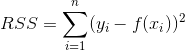•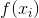是我们训练的模型：`model.predict(X_test)`
•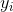是测试数据的`y``y_test`
•是指数：`**2`
•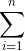是求和：`.sum()`

``````# 将我们使用训练数据创建的模型
((y_test - model.predict(X_test)) **2).sum()

# 313.6087355571951
``````

``````# 计算 MSE
np.mean((model.predict(X_test) - y_test) **2)

# 10.45362451857317
``````

## Sklearn 线性回归

``````# 加载库
from sklearn.linear_model import LinearRegression
import warnings

# 屏蔽警告
warnings.filterwarnings(action="ignore", module="scipy", message="^internal gelsd")

# 加载数据
X = boston.data
y = boston.target

# 创建线性回归
regr = LinearRegression()

# 拟合线性回归
model = regr.fit(X, y)

# 查看截距（偏差）
model.intercept_

# 36.491103280361038

# 查看特征系数（权重）
model.coef_

'''
array([ -1.07170557e-01,   4.63952195e-02,   2.08602395e-02,
2.68856140e+00,  -1.77957587e+01,   3.80475246e+00,
7.51061703e-04,  -1.47575880e+00,   3.05655038e-01,
-1.23293463e-02,  -9.53463555e-01,   9.39251272e-03,
-5.25466633e-01])
'''
``````

# 岭回归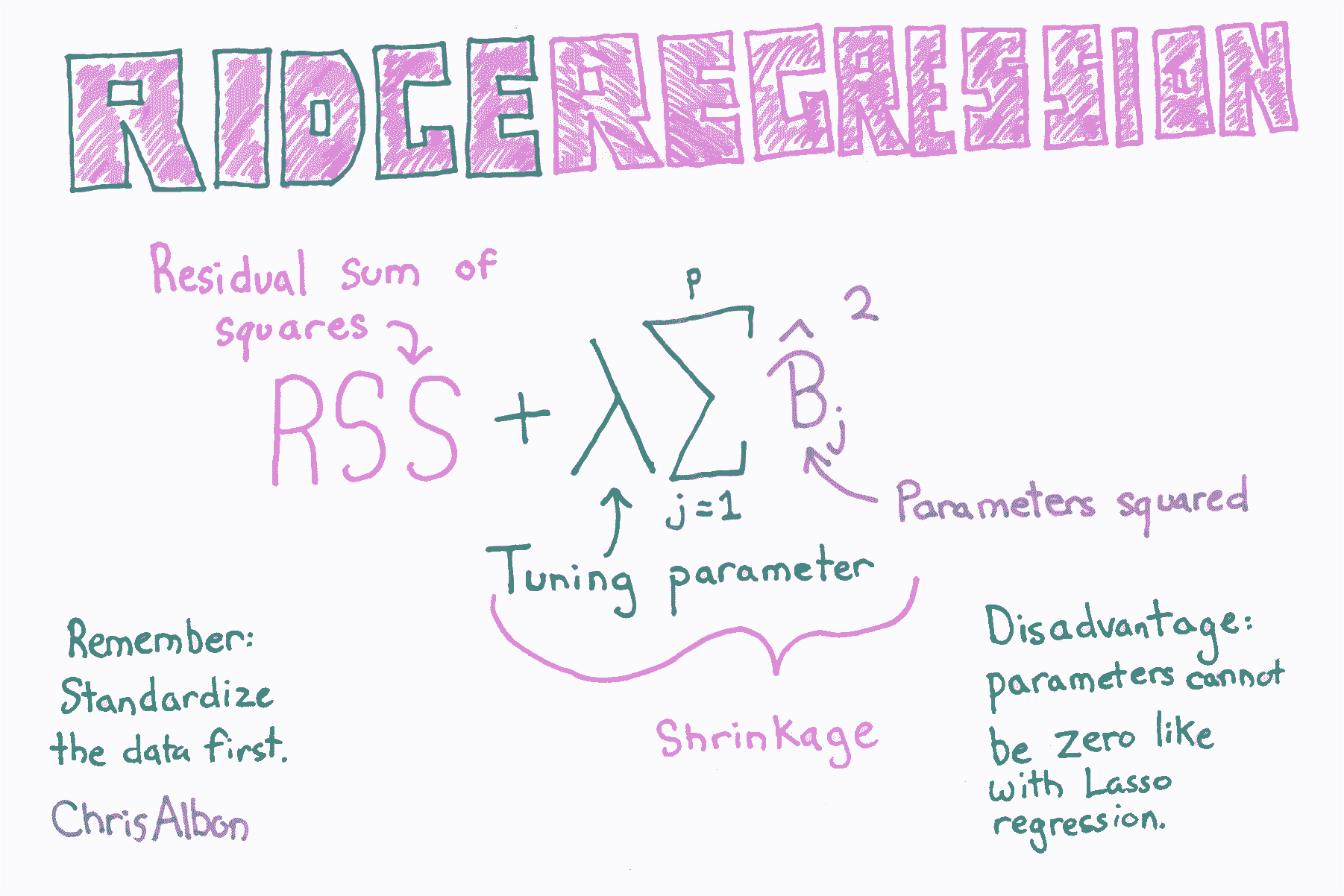``````# 加载库
from sklearn.linear_model import Ridge
from sklearn.preprocessing import StandardScaler

# 加载数据
X = boston.data
y = boston.target

# 标准化特征
scaler = StandardScaler()
X_std = scaler.fit_transform(X)
``````

``````# 创建带有 alpha 值的岭回归
regr = Ridge(alpha=0.5)

# 拟合岭回归
model = regr.fit(X_std, y)
``````

# 为岭回归选择最佳的 alpha 值

``````# 加载库
from sklearn.linear_model import RidgeCV
from sklearn.preprocessing import StandardScaler

# 加载数据
X = boston.data
y = boston.target
``````

``````# 标准化特征
scaler = StandardScaler()
X_std = scaler.fit_transform(X)

# 创建带有三个可能 alpha 值的岭回归
regr_cv = RidgeCV(alphas=[0.1, 1.0, 10.0])
``````

scikit-learn 包含`RidgeCV`方法，允许我们为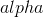选择理想值：

``````# 拟合岭回归
model_cv = regr_cv.fit(X_std, y)

# 查看 alpha
model_cv.alpha_

# 1.0
``````